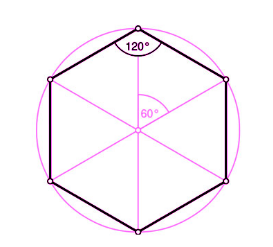# Perimeter of Hexagon Formula

In geometry, a hexagon is said to the polygon which has six sides and six angles. Hexa comes from the Greek word “Hex” meaning “six” in English and “gonia” meaning angles. If all the six sides are equal, then it is called a regular hexagon. Naturally, the perimeter of the regular hexagon will be 6 multiplied by one side of the hexagon. Each internal angle of the hexagon is

$$\begin{array}{l}120^{\circ}\end{array}$$
.Formula of Perimeter of Hexagon:

$\large P=6\times a$

Where,

a = Length of a side

### Solved Example

Question: Find the perimeter of the regular hexagon with one side 12 cm.

Solution:

Given,
a = 12 cm

Formula of hexagon perimeter,

P = 6

$$\begin{array}{l}\times\end{array}$$
a

P = 6

$$\begin{array}{l}\times\end{array}$$
12

P = 72 cm